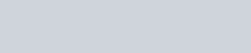# Level Order Traversal or Breadth First Traversal of a Tree

In this tutorial, we’ll be discussing what’s Level Order Traversal. Also, we’ll be printing each level of the tree. We’ll use the two major approaches – Recursive and Iterative and see what’s the difference in time complexities between them.

## Level Order Traversal

Level Order traversal is also known as Breadth-First Traversal since it traverses all the nodes at each level before going to the next level (depth).

Following illustration shows levels of a Binary Tree:The last level of the tree is always equal to the height of the tree.

The last level of the tree should contain at least one Node.

Following is the class that defines the Binary Tree Data Structure:

``````
public class BinaryTree {

public TreeNode root;

public static class TreeNode {

public TreeNode left;
public TreeNode right;
public Object data;

public TreeNode(Object data) {
this.data = data;
left = right = null;
}
}
}
``````

Let’s write the Java Program for iterative and recursive approaches for breadth-first traversal.

### Breadth First Traversal – Recursively

Following Java program uses the recursive approach to print each level of the tree:

``````
package com.journaldev.tree.levelOrderTraversal;

import com.journaldev.tree.height.BinaryTree;
import com.journaldev.tree.height.BinaryTree.TreeNode;

public class PrintLevelsOfTree {

public static void main(String[] args) {

BinaryTree binaryTree = new BinaryTree();

/**
* Binary Tree in our example, height = 2
* 		1		(Root)
* 	  2	  3		(Level 1)
*      4       5		(Level 2)
*/
binaryTree.root = new BinaryTree.TreeNode(1);
binaryTree.root.left = new BinaryTree.TreeNode(2);
binaryTree.root.right = new BinaryTree.TreeNode(3);
binaryTree.root.left.left = new BinaryTree.TreeNode(4);
binaryTree.root.right.left = new BinaryTree.TreeNode(5);

printLevelsRecursively(binaryTree.root);
}

private static void printLevelsRecursively(TreeNode root) {
int height = heightOfTree(root);

for (int i = 1; i <= height; i++) {
System.out.print("Level " + i + " : ");
printSingleLevelRecursively(root, i);
System.out.println();

}
}

private static int heightOfTree(TreeNode root) {
if (root == null) {
return 0;
}

int leftHeight = heightOfTree(root.left);
int rightHeight = heightOfTree(root.right);

return Math.max(leftHeight, rightHeight) + 1;
}

private static void printSingleLevelRecursively(TreeNode root, int level) {
if (root == null)
return;

if (level == 1) {
System.out.print(root.data + " ");
} else if (level > 1) {
printSingleLevelRecursively(root.left, level - 1);
printSingleLevelRecursively(root.right, level - 1);
}
}
}

``````

Following is the output that gets printed:Level Order Complexity for Recursive Approach

Time Complexity – O(n^2)
Space Complextiy – O(1)

Let’s optimize the above program by using the Iterative approach instead.

``````
public static void printLevelsIteratively(TreeNode root) {

while (!queue.isEmpty()) {

TreeNode node = queue.peek();
if (node != null) {
System.out.print(node.data + " ");
queue.remove();

if (node.left != null)

if (node.right != null)
}

}

}
``````

This prints the following:In the above code, each Node is enqueued and dequeued once.

Level Order Complexity for Iterative Approach

Time Complexity – O(n)
Space Complextiy – O(n)

The Queue size is equal to the number of the nodes.

So in the above approach, we’ve traded with some space for optimizing the approach.

That brings an end to this tutorial.

You can checkout complete code and more DS & Algorithm examples from our GitHub Repository.
close
Generic selectors
Exact matches only
Search in title
Search in content